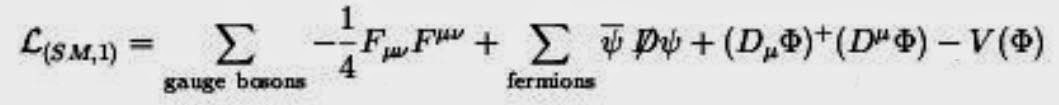### Standard Model formula (Lagrangian)

There is a type of formula that, for mathematicians and physicists, encapsulates the Standard Model. It involves a type of formula called a Lagrangian, which is crucial for particle physicists.

It came from the work of both Euler and Lagrange and, in summary, it basically calculates the system's kinetic energy minus the potential energy.

A version of the Lagrangian equation for the whole standard model is shown below. Note that the last part i.e. the V(phi) component of this equation is the term that represents the potential energy. In this case, it is very similar to the expression actually shown on Higgs' blackboard - see the previous page. It represents the [potential] energy of the system when symmetry is broken and is important for the acquisition of mass by the particles.from http://hep.ph.liv.ac.uk

In the above formula, the last term is defined as shown below. Note that the first and third "+" are not indicating addition as within each pair of phi symbols they are multiplied together. Finally, the minus symbol before the mu squared is what gives the little upward peak within the parabola called the Mexican Hat.from http://hep.ph.liv.ac.uk

Hope this has been of interest and maybe even of some help. You might like to read the article by Prof Tom Kibble (see Further Reading) if you would like lots more maths!